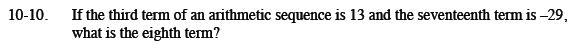Home > CCA2 > Chapter 10 > Lesson 10.1.1 > Problem10-10

10-10.

If the third term of an arithmetic sequence is 13 and the seventeenth term is −29, what is the eighth term? Homework Help ✎Create and solve a system of equations based on the sequence t(n) = an + b:

13 = a(3) + b
−29 = a(17) + b

Use the Elimination Method to solve for a and b.

t(8) = (8)(a) + b = ?